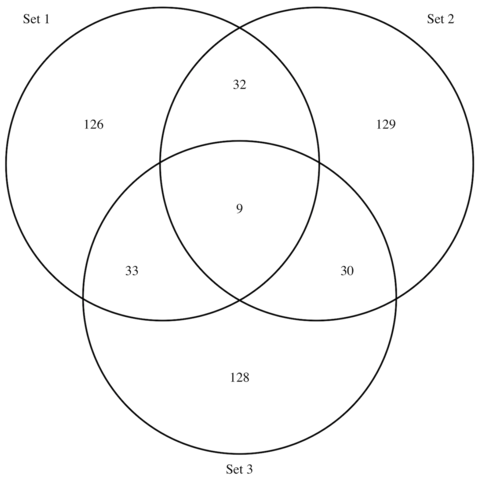# Venn Diagram

A Venn diagram shows all possible logical relationships between several sets of data. This page explains how to build one with `R` and the `VennDiagram` package, with reproducible code provided.

# Most basic

The `VennDiagram` package allows to build Venn Diagrams thanks to its `venn.diagram()` function. It takes as input a list of vector. Each vector providing words.

The function starts bycounting how many words are common between each pair of list. It then draws the result, showing each set as a circle.

The output is available as a `.png` file in your current working directory.``````# Load library
library(VennDiagram)

# Generate 3 sets of 200 words
set1 <- paste(rep("word_" , 200) , sample(c(1:1000) , 200 , replace=F) , sep="")
set2 <- paste(rep("word_" , 200) , sample(c(1:1000) , 200 , replace=F) , sep="")
set3 <- paste(rep("word_" , 200) , sample(c(1:1000) , 200 , replace=F) , sep="")

# Chart
venn.diagram(
x = list(set1, set2, set3),
category.names = c("Set 1" , "Set 2 " , "Set 3"),
filename = '#14_venn_diagramm.png',
output=TRUE
)``````

# Customize it

The `venn.diagram()` function offers several option to customize the output. Those options allow to customize the circles, the set names, and the intersection values.``````# Load library
library(VennDiagram)

# Generate 3 sets of 200 words
set1 <- paste(rep("word_" , 200) , sample(c(1:1000) , 200 , replace=F) , sep="")
set2 <- paste(rep("word_" , 200) , sample(c(1:1000) , 200 , replace=F) , sep="")
set3 <- paste(rep("word_" , 200) , sample(c(1:1000) , 200 , replace=F) , sep="")

# Prepare a palette of 3 colors with R colorbrewer:
library(RColorBrewer)
myCol <- brewer.pal(3, "Pastel2")

# Chart
venn.diagram(
x = list(set1, set2, set3),
category.names = c("Set 1" , "Set 2 " , "Set 3"),
filename = '#14_venn_diagramm.png',
output=TRUE,

# Output features
imagetype="png" ,
height = 480 ,
width = 480 ,
resolution = 300,
compression = "lzw",

# Circles
lwd = 2,
lty = 'blank',
fill = myCol,

# Numbers
cex = .6,
fontface = "bold",
fontfamily = "sans",

# Set names
cat.cex = 0.6,
cat.fontface = "bold",
cat.default.pos = "outer",
cat.pos = c(-27, 27, 135),
cat.dist = c(0.055, 0.055, 0.085),
cat.fontfamily = "sans",
rotation = 1
)``````

# Application on rap Lyrics

Here is an example showing the number of shared words in the lyrics of 3 famous french singers: (Nekfeu, Booba) and Georges Brassens.

This example is extensively described in this dedicated post.

Note the use of both the `col` and `fill` options to make the circle border color different darker.``````# Libraries
library(tidyverse)
library(hrbrthemes)
library(tm)
library(proustr)

# Load dataset from github
data <- data %>% filter(!grepl(to_remove, word)) %>% filter(!word %in% stopwords('fr')) %>% filter(!word %in% proust_stopwords()\$word)

# library
library(VennDiagram)

#Make the plot
venn.diagram(
x = list(
data %>% filter(artist=="booba") %>% select(word) %>% unlist() ,
data %>% filter(artist=="nekfeu") %>% select(word) %>% unlist() ,
data %>% filter(artist=="georges-brassens") %>% select(word) %>% unlist()
),
category.names = c("Booba (1995)" , "Nekfeu (663)" , "Brassens (471)"),
filename = 'IMG/venn.png',
output = TRUE ,
imagetype="png" ,
height = 480 ,
width = 480 ,
resolution = 300,
compression = "lzw",
lwd = 1,
col=c("#440154ff", '#21908dff', '#fde725ff'),
fill = c(alpha("#440154ff",0.3), alpha('#21908dff',0.3), alpha('#fde725ff',0.3)),
cex = 0.5,
fontfamily = "sans",
cat.cex = 0.3,
cat.default.pos = "outer",
cat.pos = c(-27, 27, 135),
cat.dist = c(0.055, 0.055, 0.085),
cat.fontfamily = "sans",
cat.col = c("#440154ff", '#21908dff', '#fde725ff'),
rotation = 1
)``````

Related chart types

## Contact

This document is a work by Yan Holtz. Any feedback is highly encouraged. You can fill an issue on Github, drop me a message on Twitter, or send an email pasting yan.holtz.data with gmail.com.